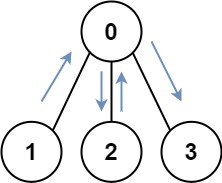## Algorithm

Problem Name: 847. Shortest Path Visiting All Nodes

You have an undirected, connected graph of `n` nodes labeled from `0` to `n - 1`. You are given an array `graph` where `graph[i]` is a list of all the nodes connected with node `i` by an edge.

Return the length of the shortest path that visits every node. You may start and stop at any node, you may revisit nodes multiple times, and you may reuse edges.

Example 1:```Input: graph = [[1,2,3],,,]
Output: 4
Explanation: One possible path is [1,0,2,0,3]
```

Example 2:```Input: graph = [,[0,2,4],[1,3,4],,[1,2]]
Output: 4
Explanation: One possible path is [0,1,4,2,3]
```

Constraints:

• `n == graph.length`
• `1 <= n <= 12`
• `0 <= graph[i].length < n`
• `graph[i]` does not contain `i`.
• If `graph[a]` contains `b`, then `graph[b]` contains `a`.
• The input graph is always connected.

## Code Examples

### #1 Code Example with Javascript Programming

```Code - Javascript Programming```

``````
const shortestPathLength = function(graph) {
const N = graph.length
const dist = Array.from({ length: 1 << N }, () => new Array(N).fill(N * N))
for (let x = 0; x < N; x++) dist[1 << x][x] = 0
for (let cover = 0; cover < 1 << N; cover++) {
let repeat = true
while (repeat) {
repeat = false
for (let next of graph[head]) {
let cover2 = cover | (1 << next)
if (d + 1 < dist[cover2][next]) {
dist[cover2][next] = d + 1
if (cover == cover2) repeat = true
}
}
}
}
}
let ans = N * N
for (let cand of dist[(1 << N) - 1]) ans = Math.min(cand, ans)
return ans
}
``````
Copy The Code &

Input

cmd
graph = [[1,2,3],,,]

Output

cmd
4

### #2 Code Example with Python Programming

```Code - Python Programming```

``````
class Solution:
def shortestPathLength(self, graph):
memo, final, q = set(), (1 << len(graph)) - 1, collections.deque([(i, 0, 1 << i) for i in range(len(graph))])
while q:
node, steps, state = q.popleft()
if state == final: return steps
for v in graph[node]:
if (state | 1 << v, v) not in memo:
q.append((v, steps + 1, state | 1 << v))
memo.add((state | 1 << v, v))
``````
Copy The Code &

Input

cmd
graph = [[1,2,3],,,]

Output

cmd
4